﻿ Appendix 6A: Bond Immunization Theoremoffice (412) 9679367
fax (412) 967-5958
toll-free 1 (800) 214-3480

D.6  Derivation of Stock Price Distribution

Let the assumed process for stock prices be described by:

.The solution to this stochastic differential equation is given bywhich implies that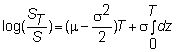Since dz is normally distributed with mean 0 and variance 1, we get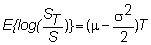The solution for ST can be verified by applying Ito's lemma to log(S).

If f(S,t) = log(S), then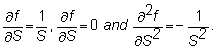Since  dS = mSdt + sSdz,  Ito's lemma yields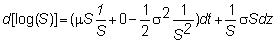so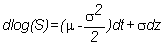Thus, log(S) is also a diffusion process, with drift  (m-s2/2) and volatility  s.

Integrating from 0 to T yields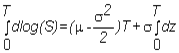This means that the change in log(S) between time 0 and time T, log(ST)-log(S), is given by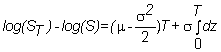so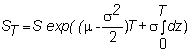This completes our characterization of the distributional properties implied from the geometric Brownian motion model for stock prices.